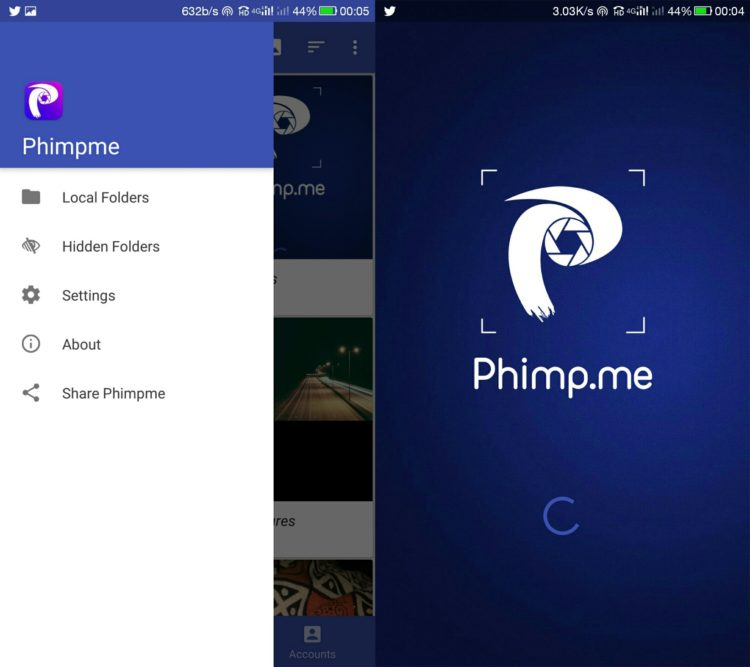# Combining multiple images into one image in the Phimpme Android Application

The Phimpme app comes with a lot of image editing functionalities. One such fascinating feature Phimpme provides is to combine two or more multiple images into one with just a button click. To do this in the Phimpme Application, long click two or more medias and click the affix button to combine them into one from the menu options. This is how two images when affixed looks like:In this post, I will talk about how to achieve this functionality in any Android application with some code snippets. Here is the step by step tutorial on how to achieve this:

Step 1:

The first step is to reduce the bitmap of the selected images to a smaller size to avoid the OutOFMemory Exception while affixing images. As by affixing the images we will be making the images smaller, we don’t need the high quality bitmaps for it. To reduce the bitmaps use the function provided below:

``````private Bitmap getBitmap(String path) {

Uri uri = Uri.fromFile(new File(path));
InputStream in = null;
try {
final int IMAGE_MAX_SIZE = 1200000; // 1.2MP
in = getContentResolver().openInputStream(uri);

// Decode image size
BitmapFactory.Options o = new BitmapFactory.Options();
o.inJustDecodeBounds = true;
BitmapFactory.decodeStream(in, null, o);
in.close();

int scale = 1;
while ((o.outWidth * o.outHeight) * (1 / Math.pow(scale, 2)) >
IMAGE_MAX_SIZE) {
scale++;
}

Bitmap bitmap = null;
in = getContentResolver().openInputStream(uri);
if (scale > 1) {
scale--;
// scale to max possible inSampleSize that still yields an image
// larger than target
o = new BitmapFactory.Options();
o.inSampleSize = scale;
bitmap = BitmapFactory.decodeStream(in, null, o);

// resize to desired dimensions
int height = bitmap.getHeight();
int width = bitmap.getWidth();

double y = Math.sqrt(IMAGE_MAX_SIZE
/ (((double) width) / height));
double x = (y / height) * width;

Bitmap scaledBitmap = Bitmap.createScaledBitmap(bitmap, (int) x,
(int) y, true);
bitmap.recycle();
bitmap = scaledBitmap;

System.gc();
} else {
bitmap = BitmapFactory.decodeStream(in);
}
in.close();

Log.d(TAG, "bitmap size - width: " +bitmap.getWidth() + ", height: " +
bitmap.getHeight());
return bitmap;
} catch (IOException e) {
Log.e(TAG, e.getMessage(),e);
return null;
}
}``````

Step 2:

Create a bitmap array of the selected images using the following snippet:

``````for (int i = 0; i < selectedMedias.size(); i++) {
}``````

Here the selectedMedias is the ArrayList<Media> files which is used to create a bitmap array of reduced pixels using the function getBitmap we created in the first step.

Step 3:

Create a union bitmap of the two images of particular width and height and then draw your bitmaps into a canvas using the Android Canvas class :

``````unionBitmap = Bitmap.createBitmap(getBitmapsWidth(bitmapArray), getMaxBitmapHeight(bitmapArray), Bitmap.Config.ARGB_8888);
Canvas comboImage = new Canvas(unionBitmap);
``````

Step 4:

The last step is to combine the two images and get the affixed Canvas as the result. This can be done using the function given below which takes the Canvas and ArrayList of bitmaps as a parameter and returns a Canvas of images.

``````private static Canvas combineBitmap(Canvas cs, ArrayList bpmList){
int width = bpmList.get(0).getWidth();
cs.drawBitmap(bpmList.get(0), 0f, 0f, null);

for (int i = 1; i < bpmList.size(); i++) {
cs.drawBitmap(bpmList.get(i), width, 0f, null);
width += bpmList.get(i).getWidth();
}
return cs;
}
``````

This is how we achieved the affixing images feature in our phimpme Android application. For the complete working source code. Refer to the Affix.java class in Phimpme Android project source code.

Resources: